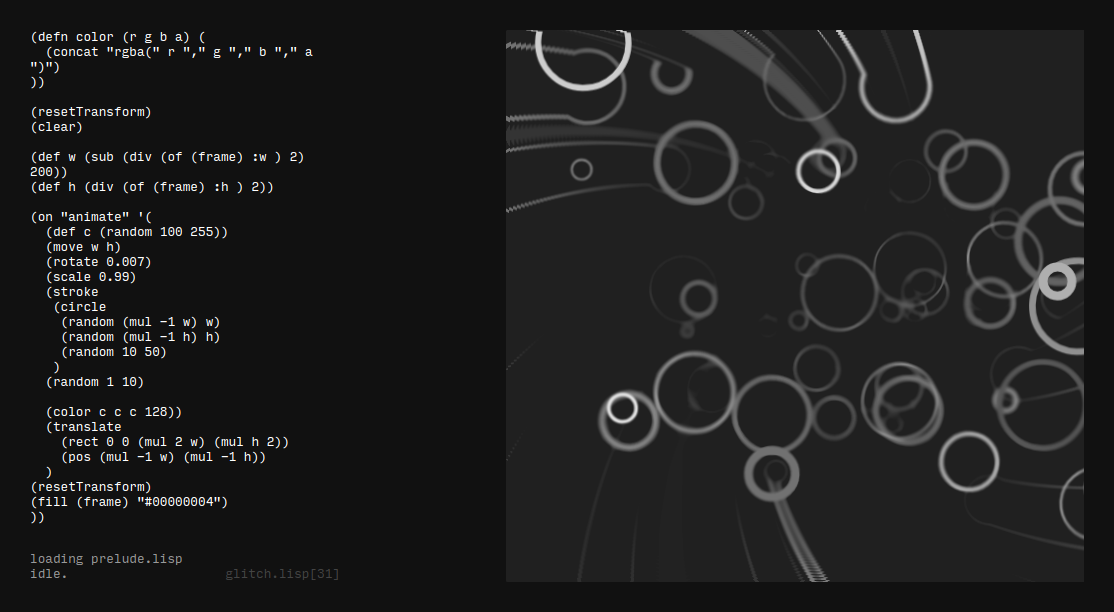## ronin experiment 8 - pixel transformation vortex

for this ronin experiment I combined the pixel transformation functions with the animation event handler to scale and rotate the previous frame. this creates a rotating vortex and everything drawn on the screen is sliding into the void slowlythis is the code i used to create the effect:

``````(defn color (r g b a) (
(concat "rgba(" r "," g "," b "," a ")")
))

(resetTransform)
(clear)

(def w (sub (div (of (frame) :w ) 2) 200))
(def h (div (of (frame) :h ) 2))

(on "animate" '(
(def c (random 100 255))
(move w h)
(rotate 0.007)
(scale 0.99)
(stroke
(circle
(random (mul -1 w) w)
(random (mul -1 h) h)
(random 10 50)
)
(random 1 10)
(color c c c 128))
(translate
(rect 0 0 (mul 2 w) (mul h 2))
(pos (mul -1 w) (mul -1 h))
)
(resetTransform)
(fill (frame) "#00000004")
))
``````